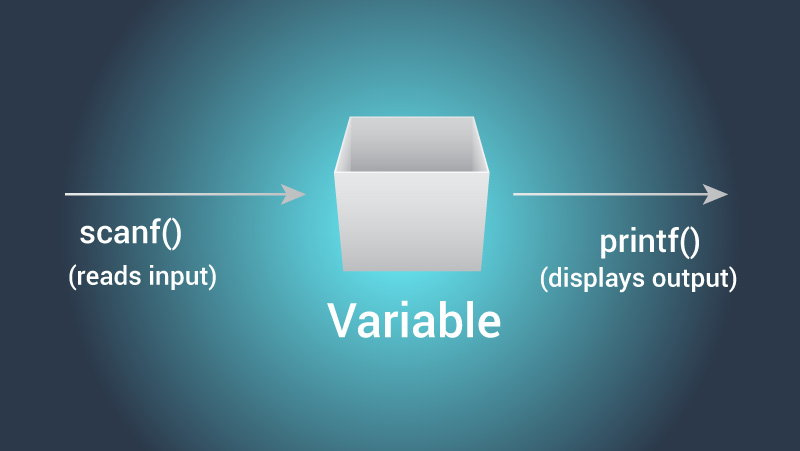# C Program to Print an Integer (Entered by the User)

In this example, the integer entered by the user is stored in a variable and printed on the screen.To understand this example, you should have the knowledge of the following C programming topics:

## Program to Print an Integer

``````#include <stdio.h>
int main() {
int number;

printf("Enter an integer: ");

scanf("%d", &number);

// displays output
printf("You entered: %d", number);

return 0;
}
``````

Output

```Enter an integer: 25
You entered: 25
```

In this program, an integer variable number is declared.

``````int number;
``````

Then, the user is asked to enter an integer number. This number is stored in the number variable.

``````printf("Enter an integer: ");
scanf("%d", &number);
``````

Finally, the value stored in number is displayed on the screen using `printf()`.

``````printf("You entered: %d", number);
``````